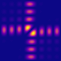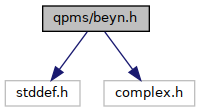QPMS Electromagnetic multiple scattering library and toolkit.
beyn.h File Reference

Beyn's algorithm for nonlinear eigenvalue problems. More...

#include <stddef.h>
#include <complex.h>
Include dependency graph for beyn.h:Go to the source code of this file.

## Data Structures

struct  beyn_contour_t
Complex plane integration contour structure. More...

struct  beyn_result_t
Beyn algorithm result structure (pure C array version). More...

## Typedefs

typedef int(* beyn_function_M_t) (complex double *target_M, size_t m, complex double z, void *params)
User-supplied function that provides the (row-major) m × m matrix M(z) whose "roots" are to be found. More...

typedef int(* beyn_function_M_inv_Vhat_t) (complex double *target_M_inv_Vhat, size_t m, size_t l, const complex double *Vhat, complex double z, void *params)
(optional) User-supplied function that, given $$\hat V$$, calculates $$M(z)^{-1} \hat V$$. More...

typedef struct beyn_contour_t beyn_contour_t
Complex plane integration contour structure.

typedef struct beyn_result_t beyn_result_t
Beyn algorithm result structure (pure C array version).

## Enumerations

enum  beyn_contour_halfellipse_orientation { BEYN_CONTOUR_HALFELLIPSE_RE_PLUS = 3 , BEYN_CONTOUR_HALFELLIPSE_RE_MINUS = 1 , BEYN_CONTOUR_HALFELLIPSE_IM_PLUS = 0 , BEYN_CONTOUR_HALFELLIPSE_IM_MINUS = 2 }

## Functions

beyn_contour_tbeyn_contour_ellipse (complex double centre, double halfax_re, double halfax_im, size_t npoints)
Complex plane elliptic integration contour with axes parallel to the real, imaginary axes. More...

beyn_contour_tbeyn_contour_halfellipse (complex double centre, double halfax_re, double halfax_im, size_t npoints, beyn_contour_halfellipse_orientation or)
Complex plane "half-elliptic" integration contour with axes parallel to the real, imaginary axes. More...

beyn_contour_tbeyn_contour_kidney (complex double centre, double halfax_re, double halfax_im, double rounding, size_t n, beyn_contour_halfellipse_orientation or)
Similar to halfellipse but with rounded corners. More...

void beyn_result_free (beyn_result_t *result)

beyn_result_tbeyn_solve (size_t m, size_t l, beyn_function_M_t M, beyn_function_M_inv_Vhat_t M_inv_Vhat, void *params, const beyn_contour_t *contour, double rank_tol, size_t rank_min_sel, double res_tol)
Solve a non-linear eigenproblem using Beyn's algorithm. More...

## Detailed Description

Beyn's algorithm for nonlinear eigenvalue problems.

## ◆ beyn_function_M_inv_Vhat_t

 typedef int(* beyn_function_M_inv_Vhat_t) (complex double *target_M_inv_Vhat, size_t m, size_t l, const complex double *Vhat, complex double z, void *params)

(optional) User-supplied function that, given $$\hat V$$, calculates $$M(z)^{-1} \hat V$$.

Pure C array version

## ◆ beyn_function_M_t

 typedef int(* beyn_function_M_t) (complex double *target_M, size_t m, complex double z, void *params)

User-supplied function that provides the (row-major) m × m matrix M(z) whose "roots" are to be found.

Pure C array version

## ◆ beyn_contour_ellipse()

 beyn_contour_t* beyn_contour_ellipse ( complex double centre, double halfax_re, double halfax_im, size_t npoints )

Complex plane elliptic integration contour with axes parallel to the real, imaginary axes.

Free using free().

## ◆ beyn_contour_halfellipse()

 beyn_contour_t* beyn_contour_halfellipse ( complex double centre, double halfax_re, double halfax_im, size_t npoints, beyn_contour_halfellipse_orientation or )

Complex plane "half-elliptic" integration contour with axes parallel to the real, imaginary axes.

Free using free().

## ◆ beyn_contour_kidney()

 beyn_contour_t* beyn_contour_kidney ( complex double centre, double halfax_re, double halfax_im, double rounding, size_t n, beyn_contour_halfellipse_orientation or )

Similar to halfellipse but with rounded corners.

Parameters
 rounding Must be in interval [0, 0.5)

## ◆ beyn_solve()

 beyn_result_t* beyn_solve ( size_t m, size_t l, beyn_function_M_t M, beyn_function_M_inv_Vhat_t M_inv_Vhat, void * params, const beyn_contour_t * contour, double rank_tol, size_t rank_min_sel, double res_tol )

Solve a non-linear eigenproblem using Beyn's algorithm.

Parameters
 m Dimension of the matrix M. l Number of columns of the random matrix $$\hat V$$ (larger than the expected number of solutions). M Function providing the matrix $$M(z)$$. M_inv_Vhat Fuction providing the matrix $$M^{-1}(z) \hat V$$ (optional). params Parameter pointer passed to M() and M_inv_Vhat(). contour Integration contour. rank_tol (default: 1e-4) TODO DOC. rank_min_sel Minimum number of eigenvalue candidates, even if they don't pass rank_tol. res_tol (default: 0.0) TODO DOC.